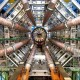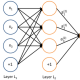Lectures

Lecture 25, April 11th, 2016: More Generative Neural Nets

This is the last lecture. You are encouraged to ask questions about anything we have discussed during the term in order to better prepare for the exam.

Please also study the following material in preparation for the class:

• End of Chapter 20 (sec. 20.11 to 20.15) of the Deep Learning Textbook (deep generative models).
• Slides on deep generative modeling (42 to 59)
Standard

25 thoughts on “Lecture 25, April 11th, 2016: More Generative Neural Nets”

1.assyatrofimov says:

I’m not sure I understand correctly the 2014 exam question #8.
Here it is:
In the case of a fully observed Boltzmann machine (no hidden units), do you believe
it is possible to take advantage of the fact that all variables are observed in order to
avoid the need for sampling negative examples (which would typically from a Markov

Here is where I get lost:
We don’t need to get negative samples since all units are visible and probabilities are accessible.
So then what does Boltzmann Machine really bring to the table?

Like

•ift6266benjamin says:

Yes, even when your Boltzmann machine is fully visible, you still need a negative phase.

The probability of a training example x is given by$P(x) = e^{-E(x)} / Z$.
Since we assume no hidden units, computing the numerator $e^{-E(x)}$ is straightforward: you get the “positive term” for free.

But the denominator (i.e. the “negative term”) is not accessible. You still need to compute the partition function Z. Hence the need for the negative phase to estimate the partition function.

Liked by 1 person

•Olexa Bilaniuk says:

At our study session I argued just the opposite. Unless I’m mistaken in my derivations, a fully visible Boltzmann machine has an energy function exactly identical to the exponent term of a general multivariate Gaussian. Whence it follows that the parameters can be gotten analytically by computing the mean and covariance of that Gaussian.

Like

•Olexa Bilaniuk says:

Amendment: A fully visible Boltzmann machine without hiddens is comparable to a multivariate Gaussian.

Like

•ryanlowe says:

Based on what Yoshua said in class: both Olexa and Benjamin are right. However, the Boltzmann machine only has the energy of a multivariate Gaussian under certain conditions (I believe if x spans the whole real line). So, in general it is true that you still need the negative phase

Like

•ryanlowe says:

Also, it was mentioned that the Gaussian equivalence holds regardless of whether there are hidden units. But these Gaussian Boltzmann machines are uninteresting, because they are basically independent of depth (it’s just a reparametrization)

Like

2.thenuttynetter says:

“Give an argument showing how a distributed representation (e.g. with an
RBM) can be up to exponentially more efficient (in terms of number of parameters vs
number of examples required) than a local representation (e.g. k-means clustering).”

Is there any particular argument that you’d recommend here? For example, are there any proofs that are simple or concise enough to put as answers for the exam?

Like

•tisu32 says:

The classical argument (making abstraction of the type of representation chosen) would be representing 2^n examples as either:
– distributed: a vector in {0,1}^n, number of parameters n
– local: one-hot encoding on the set {0,1}^n, requiring 2^n parameters.

To give a practical example: we want to track presence or absence (1 or 0) of n genes. A distributed representation would be a vector of length n, with 0s and 1s at the corresponding index of the gene. A local representation would be an example (cluster) which would live in a space with 2^n possibilities.

Liked by 1 person

•christopherjbeckham says:

To add another view, we can think of the distributed representation as providing better “statistical efficiency” — for a one-hot vector, you will need$N$ params for$N$ examples (in this example let a “param” be a binary hidden unit). For a distributed representation, you will only need$log2(N)$ params for$N$ examples, e.g., to represent 64 examples, we need a hidden unit vector of size$log2(64) = 6$ (since$2^6 = 64$).

Liked by 1 person

•ryanlowe says:

To add to this, there is also the ‘no free lunch principle’, which tells us that this exponential efficiency does not hold for all functions we want to approximate; indeed, for most randomly drawn functions, it will not be the case. But ‘real world data’ seems to be fundamentally compositional in nature, and is thus amenable to these speedups

Like

•etienneift6266 says:

I’m not sure whether or not this would be sufficient (or if it is even right), but as we saw in last lecture, an RBM has n, number of visible units (dimensions of x), time m, number of hidden units, parameters and can represent 2^m mode. On the other hand, local representations need to “remember” every training examples (so O(n*N) parameters) to represent N mode (N is the number of training examples). For (nearly) any choice of n and N, there will always be a m that is such that the RBM has more modes while having less parameters. One only needs 2^m > N and m<N.

Like

3.Vincent says:

From the H13 final :

” Use a Taylor expansion to show that the training criterion of a denoising auto-encoder (with Gaussian noise N ~ (0;$\sigma^2$) and quadratic reconstruction loss), is equivalent to the training criterion of a particular contractive auto-encoder when$\sigma$ goes to 0 (but where the contraction penalty is applied on the whole auto-encoder and not the encode only. ”

I am clueless. Do you have insight on how to prove that?

Like

•tisu32 says:$L = E_{p, \epsilon \equiv N(0,\sigma^2)} || x - g \circ f (x + \epsilon) ||^2 = E_{p, \epsilon \equiv N(0,\sigma^2)} || x - g \circ f (x ) + (g \ circ f)'(x)\epsilon) ||^2 = E_{p, \epsilon \equiv N(0,\sigma^2)} || x - g \circ f (x ) || ^2 + ||J_(g \ circ f)(x)\epsilon ||^2 + 2 * \epsilon$

The last term has expectancy 0.

Like

•tisu32 says:

Hmmm I can’t seem to get latex to work on wordpress, but you get the idea.

Like

•tisu32 says:

Also I forgot to mention that the second term, since \epsilon and the Jacobian are independent, you can separate into two products and take the expectancy. The expectancy of norm of \epsilon squared is sigma*2 and the first term is the Jacobian we want.

Like

•Vincent says:

Thank you much ! To get latex working, write “latex” after the first dollar sign.

Like

4.Jonathan says:

In RNN teacher forcing techniques, we sometimes connect the model output o_t to the next time step’s hidden layer h_(t+1) and sometimes we connect the correct output y_t to the next time step’s hidden layer h_(t+1). Has anyone tried to add a discriminator network to distinguish between samples drawn from training data y_t and generator data z_t, using the prob that x_t is a real training example d(x_t;θt) as the input to h(t+1)?

Like

•Dzmitry Bahdanau says:

To add to Yoshua’s answer, one possible challenge with Recurrent GAN’s is that the sequences that RNN handle often consist of discrete elements, and doing GAN with discrete generated variable is likely to be quite challenging.

Liked by 1 person

•Jonathan says:

Thanks. Let me know if there are interesting papers on the subject.

Like

5.Olivier Mastropietro says:

In H15 exam it is asked which model between VAE and DBM use parametric or non-parametric variational inference. I can’t recall where we saw those definitions so I am unsure what would be the answer here.

Like

•Dzmitry Bahdanau says:

I am not sure how much real Bayesians would like using terms “non-parameteric” and “parametric” as they are used in the question, but from the context it is quite clear what the author of the question meant.

In standard variational inference, for instance in the iterative mean-field method used in DBM, approximate inference is an algorithm for tuning the parameters of the approximate posterior to minimize the divergence between this approximate posterior and the true one. This algorithm is fixed, it does not have its own parameters. On the other hand, approximate inference can be a parametric function, that given the values of visible units output the parameters of the approximate posterior. This is the kind of inference that we have in VAE. It seems that the right answer to the question is to call VAE-like inference parametric, as opposed to the standard variational inference. The latter can be called non-parametric, due to the fact that the inference algorithm does not have its own parameters (not be confused with the parameters of the approximate posterior that it produces).

Like

6.Yifan Nie says:

Bonjour/Hi,
I have a general question about the Convolutional Boltzmann Machine mentioned in Section 20.6 of the deep learning book. I saw that this model is more complex to train that an CNN, or a simple Boltzmann Machine. And I saw that for image based applications, CNN is still very popular. What’s the advantages of this Convolutional Boltzmann Machine compared to a traditional CNN, will the performance gain worth the more difficult training compared to a normal CNN? Is there any empirical study comparing the 2 models? Thanks a lot.

Liked by 1 person

•thenuttynetter says:

To my knowledge RBMs currently don’t have state-of-the-art empirical results on any tasks.

I think that the most interesting property of RBMs is that they have a consistent generation model p(x | h) and inference model p(h | x) which correspond to the same joint p(x, h). On the other hand, models like variational autoencoders have to learn an approximate inference model q(h | x), which may not be consistent with the generation model p(x | z).

Like

7.Yifan Nie says:

Bonjour/Hi
I have a question about exam H12, in exercice 2 it is asked How could learning of deep representations be useful to perform transfer (e.g. where most examples are from classes other than the classes of interest)? Is that because we have trained share intermediate representations that can be used to perform another task? Thanks a lot .

Like

•Olexa Bilaniuk says:

I think so too. I’d add that shallow architectures learn features that are less reusable because they’re specifically aimed at the class they’re designed for (They must, since there are so few layers). Deep architectures learn a hierarchy of composable features, with only the higher-level ones characterizing the class. A transfer learning task can then automatically decide which features at which level of the hierarchy to use, with less similar objects sharing only lower-level features.

Liked by 1 person